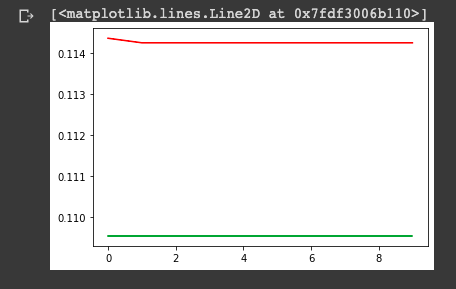# Plotting ROC curve in R Programming

• Last Updated : 01 May, 2022

Error metrics allow us to evaluate and justify the model’s performance on a specific dataset. One such error metric is the ROC plot. A classification error metric is the ROC plot, also known as the ROC AUC curve. That is, it assesses the performance and outcomes of classification machine learning algorithms.

To be more specific, the ROC curve represents the value’s probability curve, whereas the AUC is a measure of the separability of different groups of values/labels. The ROC AUC curve can be used to analyze and draw conclusions about how many values have been correctly distinguished and classified by the model based on the labels.

The higher the AUC score, the better the prediction of the predicted values. In technical terms, the ROC curve is the relationship between a model’s True Positive Rate and False Positive Rate. Let us now try to apply the concept of the ROC curve in the following section.

## Method 1: Using the plot() function

As previously discussed, we can use ROC plots to evaluate Machine Learning models. So, let us try applying the ROC curve concept to the Logistic Regression model.

In this example, we would model the Bank Loan Defaulter dataset using Logistic Regression. The ROC curve would be plotted using the plot() function from the ‘pROC’ library. The dataset can be found here!

• First, we use the read.csv() function to load the dataset into the environment.
• Prior to modelling, it is critical to split the dataset. As a result, we sample the dataset into training and test data values using the R documentation’s createDataPartition() function.
• To assess the model’s performance, we established error metrics such as Precision, Recall, Accuracy, F1 score, ROC plot, and so on.
• After then, we apply Logistic Regression to our dataset using the R glm() function. The model is then tested on the testing data using the predict() function, and the error metrics are calculated.
• Finally, we compute the roc AUC score for the model using the roc() method and plot it using the plot() function from the ‘pROC’ library.

Link to the used CSV file: bank-loan.

## R

 `rm``(list=``ls``())`` ` `# Setting the working directory``setwd``(``"D:/Edwisor_Project - Loan_Defaulter/"``)``getwd``()`` ` `# Load the dataset``gfgDataset = ``read.csv``(``"bank-loan.csv"``, header=``TRUE``)`` ` `### Data SAMPLING ####``library``(caret)``set.seed``(101)``split = ``createDataPartition``(data\$default, p=0.81, list=``FALSE``)``train_data = data[split, ]``test_data = data[-split, ]`` ` `# error metrics -- Confusion Matrix``err_metric = ``function``(GFGCM)``{``    ``GFGTN = GFGCM[1, 1]``    ``GFGRATE = GFGCM[2, 2]``    ``FP = GFGCM[1, 2]``    ``FN = GFGCM[2, 1]``    ``gfgPrecise = (GFGRATE)/(GFGRATE+FP)``    ``recall_score = (FP)/(FP+GFGTN)``    ``f1_score = 2*((gfgPrecise*recall_score)/(gfgPrecise+recall_score))``    ``accuracy_model = (GFGRATE+GFGTN)/(GFGRATE+GFGTN+FP+FN)``    ``False_positive_rate = (FP)/(FP+GFGTN)``    ``False_negative_rate = (FN)/(FN+GFGRATE)``    ``print``(``paste``(``"GfgPrecise value of the model: "``, ``round``(gfgPrecise, 2)))``    ``print``(``paste``(``"Accuracy of the model: "``, ``round``(accuracy_model, 2)))``    ``print``(``paste``(``"Recall value of the model: "``, ``round``(recall_score, 2)))``    ``print``(``paste``(``"False Positive rate of the model: "``, ``round``(False_positive_rate, 2)))``    ``print``(``paste``(``"False Negative rate of the model: "``, ``round``(False_negative_rate, 2)))``    ``print``(``paste``(``"f1 score of the model: "``, ``round``(f1_score, 2)))``}`` ` `# 1. Logistic regression``logit_m = ``glm``(formula=default~., data=train_data, family=``'binomial'``)``summary``(logit_m)``logit_P = ``predict``(logit_m, newdata=test_data[-13], type=``'response'``)``logit_P < - ``ifelse``(logit_P > 0.5, 1, 0)  ``# Probability check``GFGCM = ``table``(test_data[, 13], logit_P)``print``(GFGCM)``err_metric``(GFGCM)`` ` `# ROC-curve using pROC library``library``(pROC)``roc_score = ``roc``(test_data[, 13], logit_P)  ``# AUC score``plot``(roc_score, main=``"ROC curve -- Logistic Regression "``)`## Method 2: Using of the roc.plot() function

To plot the ROC-AUC curve for a model, we can use another library called verification in R programming. To use the function, we must first install and import the verification library into our environment.

After that, we plot the data using the roc.plot() function to get a clear picture of the ‘Sensitivity’ and ‘Specificity’ of the data values, as shown below.

## R

 `install.packages``(``"starter"``)`` ` `library``(verification)``x<- ``c``(0,0,0,1,0,0)``y<- ``c``(.7, .7, 0, 1,5,.6)`` ` `gfgData<-``gfgData.frame``(x,y)``names``(data)<-``c``(``"plot"``,``"axis"``)``roc.plot``(gfgData\$yes, gfgData\$no)`My Personal Notes arrow_drop_up